1. /
2. CBSE
3. /
4. Class 12
5. /
6. Physics
7. /
8. NCERT Solutions class 12...

# NCERT Solutions class 12 physics Current Electricity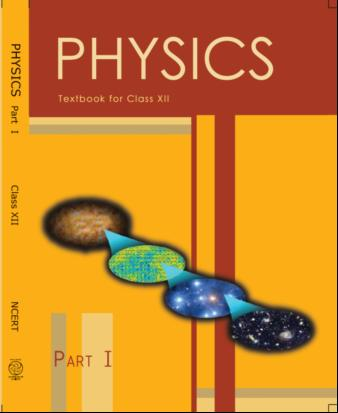## NCERT Class 12 Physics Chapter-wise Solutions

• 1 – Electric Charges and Fields
• 2 – Electrostatic Potential and Capacitance
• 3 – Current Electricity
• 4 – Moving Charges and Magnetism
• 5 – Magnetism and Matter
• 6 – Electromagnetic Induction
• 7 – Alternating Current
• 8 – Electromagnetic Waves
• 9 – Ray Optics and Optical Instruments
• 10 – Wave Optics
• 11 – Dual Nature of Radiation and Matter
• 12 – Atoms
• 13 – Nuclei
• 14 – Semiconductor Electronic: Material, Devices and Simple Circuits
• 15 – Communication Systems

## CHAPTER 3 CURRENT ELECTRICITY

• 3.1 Introduction
• 3.2 Electric Current
• 3.3 Electric Currents in Conductors
• 3.4 Ohm’s law
• 3.5 Drift of Electrons and the Origin of Resistivity
• 3.6 Limitations of Ohm’s Law
• 3.7 Resistivity of various Materials
• 3.8 Temperature Dependence of Resistivity
• 3.9 Electrical Energy, Power
• 3.10 Combination of Resistors — Series and Parallel
• 3.11 Cells, emf, Internal Resistance
• 3.12 Cells in Series and in Parallel
• 3.13 Kirchhoff’s Laws
• 3.14 Wheatstone Bridge
• 3.15 Meter Bridge
• 3.16 Potentiometer

## NCERT Solutions Class 12 physics Current Electricity

1: The storage battery of a car has an emf of 12 V. If the internal resistance of the battery is 0.4,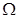what is the maximum current that can be drawn from the battery?

2: A battery of emf 10 V and internal resistance 3is connected resistor. If the current in the circuit is 0.5 A, what is the resistance of the resistor? What is the terminal voltage of the battery when the circuit is closed?

3: (a) Three resistors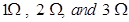are combined in series. What is the total resistance of the combination?

(b) If the combination is connected to a battery of emf 12 V and negligible internal resistance, obtain the potential drop across each resistor.

4. (a) Three resistors 2 Ω, 4 Ω and 5 Ω are combined in parallel. What is the total resistance of the combination?

(b) If the combination is connected to a battery of emf 20 V and negligible internal resistance, determine the current through each resistor, and the total current drawn from the battery.

5: At room temperature (27.0 °C) the resistance of a heating element is 100 Ω. What is the temperature of the element if the resistance is found to be 117 Ω, given that the temperature coefficient of the material of the resistor is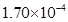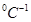?

6: A negligibly small current is passed through a wire of length 15 m and uniform cross section 6.0 ×, and its resistance is measured to be 5.0. What is the material at the temperature of the experiment?

8: A heating element using nichrome connected to a 230 V supply draws an initial current of 3.2 A which settles after a few seconds toa steady value of 2.8 A. What is the steady temperature of the heating element if the room temperature is 27.0 °C? Temperature coefficient of resistance of nichrome averaged over the temperature range involved is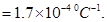9: Determine the current in each branch of the network shown in fig 3.30:10: (a) In a metre bridge [Fig. 3.27], the balance point is found to be at 39.5 cm from the end when the resistor is of 12.5 Ω.

(b) Determine the balance point of the bridge above if X and Y are interchanged.

(c) What happens if the galvanometer and cell are interchanged at the balance point of the bridge? Would the galvanometer show any current?

11: A storage battery of emf 8.0 V and internal resistance 0.5is being charged by a 120 V dc supply using a series resistor of 15.5. What is the terminal voltage of the battery during charging? What is the purpose of having a series resistor in the charging circuit?

12: In a potentiometer arrangement, a cell of emf 1.25 V gives a balance point at 35.0 cm length of the wire. If the cell is replaced by another cell and the balance point shifts to 63.0 cm, what is the emf of the second cell?

13: The number density of free electrons in a copper conductor estimated in Example is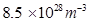. How long does an electron take to drift from one end of a wire 3.0 m long to its other end? The area of cross section of the wire is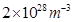and it is carrying a current of 3.0 A.

14: The earth’s surface has a negative surface charge density of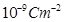. The potential difference of 400 kV between the top of the atmosphere and the surface results (due to the low conductivity of the lower atmosphere) in a current of only 1800 A over the entire globe. If there were no mechanism of sustaining atmospheric electric field, how much time (roughly) would be required to neutralize the earth’s surface? (This never happens in practice because there is a mechanism to replenish electric charges, namely the continual thunderstorms and lightning in different part of the globe [Radius of earth =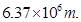15: (a) Six lead-acid type of secondary cells each of emf 2.0 V and internal resistance 0.015are joined in series to provide a supply to a resistance of 8.5. What are the current drawn from the supply and its terminal voltage?

(b) A secondary cell after long use has an emf of 1.9 V and a large internal resistance of 380 Ω. What maximum current can be drawn from the cell? Could the cell drive the starting motor of a car?

16: Two wires of equal length, one of aluminum and the other of copper have the same resistance. Which of the two wires is lighter? Hence explain why aluminum wires are preferred for overhead power cables. (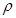Al =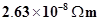Cu =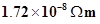, Relative density of Al = 2.7, of Cu = 8.9.)

17: What conclusion can you draw from the following observations on a resistor made of alloy manganin?

 CURRENT VOLTAGE CURRENT VOLTAGE 0.2 3.94 3 59.2 0.4 7.87 4 78.8 0.6 11.8 5 98.6 0.8 15.7 6 118.5 1.0 19.7 7 138.2 2.0 39.7 8 158.0

[a ] A steady current flows in a metallic conductor of non uniform cross section of non uniform cross section. Which of these quantities is constant along the conductor,: current ,current density ,electric field, drift speed?

[b ] Is Ohm’s law universally applicable for all conducting elements?

If not, give examples of elements which do not obey Ohm’s law.

[c] A low voltage supply from which one needs high currents must have very low resistance. Why?

[d] A high tension (HT) supply of, say, 6 kV must have a very large internal resistance

19: Choose the correct alternative:

(a) Alloys of metals usually have (greater/less) resistivity than that of their constituent metals.

(b) Alloys usually have much (lower/higher) temperature coefficients of resistance than pure metals.

(c) The resistivity of the alloy manganin is nearly independent of/increases rapidly with increase of temperature.

(d) The resistivity of a typical insulator (e.g., amber) is greater than that of a metal by a factor of the order of (1022/103).

20:  [a ] Given resistors each of resistance R, how will you combine them to get the (i) maximum (ii) minimum effective resistance? What is the ratio of the maximum to minimum resistance?

[b] Given the resistances of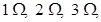how will be combine them to get an equivalent resistance of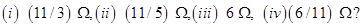[c]Determine the equivalent resistance of networks shown in fig.3.31.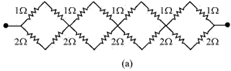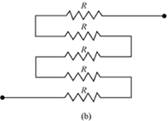21:  Determine the current drawn from a 12 V supply with internal resistance 0.5 Ω by the infinite network shown in Fig 3.32.Each resistor has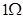resistance.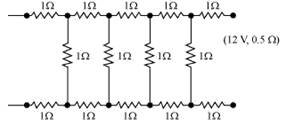22: Figure shows a potentiometer with a cell of 2.0 V and internal resistance 0.40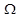maintaining a potential drop across the resistor wire AB. A standard cell which maintains a constant emf of 1.02 V (for very moderate currents up to a few mA) gives a balance point at 67.3 cm length of the wire. To ensure very low currents drawn from the standard cell, a very high resistance of 600 kΩ is put in series with it, which is shorted close to the balance point. The standard cell is then replace by a cell of unknown emf and the balance point found similarly, turns out to be at 82.3 cm length of the wire.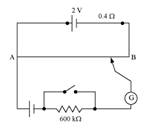[a]What is the value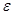?

[b]What purpose does the high resistance of 600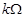have?

[c]Is the balance point affected by this high resistance?

[d]Is the balance point affected by the internal resistance of the driver cell?

[e]Would the method work in the above situation if the driver cell of the potentiometer had an emf of 1.0 V instead of 2.0 V?

[f] Would the circuit work well for determining an extremely small emf, say of the order of a few mV (such as the typical emf of a thermo-couple)? If not, how will you modify the circuit?

23: Figure 3.34 shows a potentiometer circuit for comparison of two resistances. The balance point with a standard resistor R = 10.0is found to be 58.3 cm, while that with the unknown resistance X is 68.5 cm. Determine the value of X. What might you do if you failed to find a balance point with the given cell of emf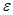?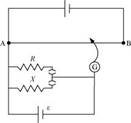24: Figure shows a 2.0 V potentiometer used for the determination of internal resistance of a 1.5 V cell. The balance point of the cell in open circuit is 76.3 cm. When a resistor of 9.5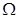is used  in the external circuit of the cell, the balance point shifts to 64.8 cm length of the potentiometer wire. Determine the internal resistance of the cell.

## NCERT Solutions for Class 12 Physics

NCERT Solutions Class 12 Physics PDF (Download) Free from myCBSEguide app and myCBSEguide website. Ncert solution class 12 physics includes textbook solutions from both part 1 and part 2. NCERT Solutions for CBSE Class 12 Physics have total 20 chapters. 12 Physics NCERT Solutions in PDF for free Download on our website. Ncert physics class 12 solutions PDF and physics ncert class 12 PDF solutions with latest modifications and as per the latest CBSE syllabus are only available in myCBSEguide

To download NCERT Solutions for class 12 Physics, Chemistry, Biology, History, Political Science, Economics, Geography, Computer Science, Home Science, Accountancy, Business Studies and Home Science; do check myCBSEguide app or website. myCBSEguide provides sample papers with solution, test papers for chapter-wise practice, NCERT solutions, NCERT Exemplar solutions, quick revision notes for ready reference, CBSE guess papers and CBSE important question papers. Sample Paper all are made available through the best app for CBSE students and myCBSEguide website.### Test Generator

Create question paper PDF and online tests with your own name & logo in minutes.### myCBSEguide

Question Bank, Mock Tests, Exam Papers, NCERT Solutions, Sample Papers, Notes